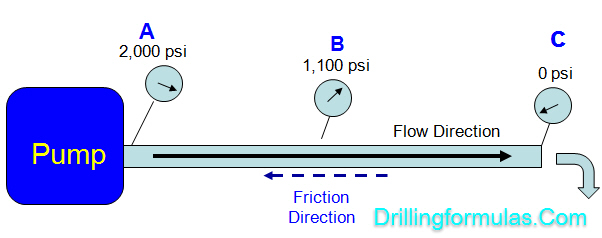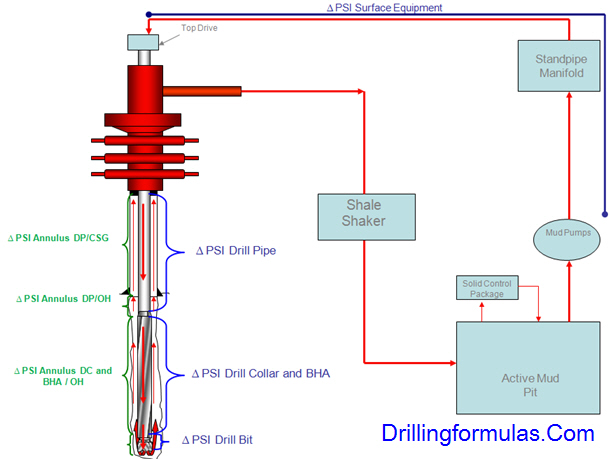# Understand Pressure Loss (Frictional Pressure) in Drilling System

Frictional pressure is pressure loss acting in the opposite direction of fluid flow and today we will look into each component in pressure therefore you will get clearer picture about the frictional pressure in drilling system.

Let take a look at the simple diagram below.A mud pump creates power to move drilling fluid from point A to C and the frictional pressure or pressure loss is the amount of pressure required to transfer fluid.

Pump pressure is 2,000 psi at the starting point (“A”) and at the end point (“C”), pressure is 0 psi. This tells you that you need 2,000 psi to overcome the frictional pressure in order to move the fluid from point “A” to point “C”.

“Differential pressure between two points in the system is pressure loss while fluid is moved from one to another point.”

What is the pressure loss from “A” and “B”?

At point “B”, the pressure remaining is 1,000 psi, therefore pressure loss between “A” and “B” equals to 900 psi (2,000 – 1,100).

What is the pressure loss from “B” and “C”?

At point “C”, at an atmospheric condition, the pressure is 0 psi, therefore pressure loss between “B” and “C” equals to 1,100 psi (1,100 – 0).

Apply This Concept Into Drilling

Then we will apply this concept to understand about pressure loss in the drilling system. The simple drawing below demonstrates a simple circulating route of drilling fluid while drilling.Analysis of Pressure Loss in The Drilling System

We need to analyze each section to understand each pressure loss component.Surface -> ∆ PSI Surface Equipment

Inside drill string, drill collar, BHA -> ∆ PSI Drill Pipe + ∆ PSI Drill Collar and BHA

Bit -> ∆ PSI Drill Bit

Annulus -> ∆ PSI Annulus DC and BHA / OH + ∆ PSI Annulus DP/OH + ∆ PSI Annulus DP/CSG

Where;

∆ PSI Surface Equipment = Pressure loss in surface equipment

∆ PSI Drill Pipe = Pressure loss in drill pipe

∆ PSI Drill Collar and BHA = Pressure loss in drillcollar and BHA

∆ PSI Drill Bit = Pressure loss across the bit

∆ PSI Annulus DC and BHA / OH = Pressure loss in the annulus between DC/BHA and open hole

∆ PSI Annulus DP/OH = Pressure loss in the annulus between drill pipe and open hole

∆ PSI Annulus DP/CSG = Pressure loss in the annulus between drill pipe and casing

In order to pump drilling fluid from the mud pit and back to surface, pump pressure equals to summation of pressure loss of all equipment which can be described in the following relationship.

Pump pressure = ∆ PSI Surface Equipment + ∆ PSI Drill Pipe + ∆ PSI Drill Collar and BHA + ∆ PSI Drill Bit ∆ PSI Annulus DC and BHA / OH + ∆ PSI Annulus DP/OH + ∆ PSI Annulus DP/CSG

Example: Well depth is 12,000’MD/10,000 TVD and planned pump rate is 400 GPM. Based on drilling hydraulics calculation, the pressure loss component s are listed below;

∆ PSI Surface Equipment =50 psi

∆ PSI Drill Pipe = 500 psi

∆ PSI Drill Collar and BHA = 30 psi

∆ PSI Drill Bit = 1500 psi

∆ PSI Annulus DC and BHA / OH = 200 psi

∆ PSI Annulus DP/OH = 600 psi

∆ PSI Annulus DP/CSG = 450 psi

What is the pump pressure required to circulate at drilling rate?

Pump pressure = total pressure loss of all equipment together.

Pump pressure = 50 + 500 + 30 + 1500 + 200 + 600 + 450 = 3,300 psi.

Note: Additional drilling formulas for hydraulic calculations that you might need to know.

Reference:Drilling Hydraulic Books

Share the joy
Tagged , . Bookmark the permalink.